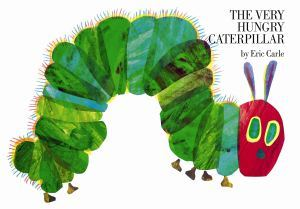# The Very Hungry Caterpillar

Alignments to Content Standards: 1.OA.A.2 1.OA.C.5 1.OA.D.7 1.NBT.B.2

#### Materials

• The Very Hungry Caterpillar by Eric CarleThe students work individually or in pairs. Each student or pair needs:

• Three ten-frames for each student or pair of students (see PDF for black line master)
• 30 counters or unifix cubes per pair of students
• One small dry-erase board and dry-erase maker per pair of students

#### Actions

The teacher reads the book to the class and asks, “How many things do you think the caterpillar ate in this story?” The students take a minute to share their estimate with a partner. Next, the teacher reads The Very Hungry Caterpillar again. After each page, the teacher pauses so that the students can add counters or unifix cubes to the ten-frame to represent the number of things the caterpillar ate, and then write an equation on the dry-erase board connecting addition to the number of counters used. After each ten-frame is filled in the students move to the next one. If the students are working in pairs, one student can add the counters/unifix cubes to the ten-frame while the other student writes the equation. By the end of the story, there should be a total of 25 food items eaten and 1 leaf eaten. (The students can decide as a class whether to count the leaf as a food). There will be two ten-frames completed with 5 or 6 counters/unifix cubes on the third ten-frame. If students come up with different, but correct, equations, then discuss the different equations and ask students, "Can all of these be correct?"

## IM Commentary

The purpose of this task is for students to solve word problems that call for addition of three whole numbers (1.OA.2), to relate counting on to addition (1.OA.5), and to understand that the two digits of a two-digit number represent amounts of tens and ones (1.NBT.2). This task supports developing conceptions of counting on and base-ten structure, and is thus appropriate early in the school year.

There is the possibility that students may write different, but correct, equations. If this happens, then the teacher should take the opportunity to ask students whether the different equations are correct and how they know. An appropriate classroom discussion can help support students' understanding of the equals sign (1.OA.7). While the standard only calls for sums within 20, in instructional situations it is appropriate to go beyond that. This limit is most salient for assessment developers.

Note that if this task is to support all these different standards, the teacher needs to be aware of the various connections and take the opportunity to draw them out as necessary.

To see an annotated version of this and other Illustrative Mathematics tasks as well as other Common Core aligned resources, visit Achieve the Core http://achievethecore.org/.

The Standards for Mathematical Practice focus on the nature of the learning experiences by attending to the thinking processes and habits of mind that students need to develop in order to attain a deep and flexible understanding of mathematics. Certain tasks lend themselves to the demonstration of specific practices by students. The practices that are observable during exploration of a task depend on how instruction unfolds in the classroom. While it is possible that tasks may be connected to several practices, only one practice connection will be discussed in depth. Possible secondary practice connections may be discussed but not in the same degree of detail.

Although this particular task can be linked to several of the Mathematical Practice Standards, the focus of this discussion will be on Mathematical Practice 7, Look for and make use of structure. First graders are building on their previous knowledge of adding smaller numbers and taking advantage of more sophisticated strategies such as “making tens” to solve addition problems. They use this structure of numbers to recognize that numbers from 11 to 19 consist of a ten and some number of ones. They notice the same holds true for the numbers 21 to 29. These numbers are composed of two tens and some number of ones. This activity links their understanding of place value structure to numbers beyond ten.

## Attached Resources

• Three ten-frames printable for classroom use
• ## Solution

An example of what the students will be doing as the story is read:

After 1 apple and 2 pears are eaten, there will be 3 counters on the ten-frame. The equation will be 1+2=3.

After 1 apple, 2 pears, and 3 plums are eaten, there will be 6 counters on the ten-frame. The equation could be either 3+3=6 or 1+2+3=6.

After 1 apple, 2 pears, 3 plums, and 4 strawberries are eaten, 4 more counters would be added to the ten-frame for a total of ten counters. The equation could be 6+4=10, 3+3+4=10, or 1+2+3+4=10.

And so on!